排序算法汇总

1.冒泡排序

``def BubbleSort(A):#冒泡，一趟最小排最前,N-1趟    if A == None or len(A) == 0:        return    for i in range(len(A)):        for j in range(len(A)-1, i, -1):            if A[j] < A[j-1]:                tmp = A[j-1]#Swap()                A[j-1] = A[j]                A[j] = tmp        #print(A)    return A``

``def BubbleSort2(A):    flag = True    for i in range(len(A)):        if flag:#为真时才执行一趟            for j in range(len(A)-1, i, -1):                flag = False                if A[j] < A[j-1]:                    tmp = A[j-1]#Swap()                    A[j-1] = A[j]                    A[j] = tmp                    flag = True#交换            #print(A)#看比较的次数    return A``

2.选择排序

``def SelectSort(A):    for i in range(len(A)):        min = i#最小值所在的位置，最小放最前        for j in range(i+1, len(A)):            if A[min] > A[j]:                min = j        if min != i:            tmp = A[min]            A[min] = A[i]            A[i] = tmp    return A``

3.插入排序

``def InsertSort(A):    for i in range(1, len(A)):#第一个默认有序        tmp = A[i]        for j in range(i-1, -1, -1):#j=i-1,j>=0,j--            if tmp < A[j]:                A[j+1] = A[j]                A[j] = tmp    return A``

4.希尔排序

``def ShellSort(A):#希尔排序/相当加了个间隔，将数据分组处理    gap = len(A) / 2    while gap >= 1:#下面就是一个插入排序过程，只是每个过程都是有间隔,j+gap        for i in range(gap, len(A)):            tmp = A[i]            j = i - gap            while j >= 0 and tmp < A[j]:                A[j+gap] = A[j]                j -= gap            A[j+gap] = tmp        gap /= 2    return A``

5.快速排序

5,3,8,6,4 用5作为比较的基准，最终会把5小的移动到5的左边，比5大的移动到5的右边。

5,3,8,6,4 首先设置i,j两个指针分别指向两端，j指针先扫描（首先这也不是绝对的，这取决于基准数的位置，因为在最后两个指针相遇的时候，要交换基准数到相遇的位置。一般选取第一个数作为基准数，那么就是在左边，所以最后相遇的数要和基准数交换，那么相遇的数一定要比基准数小。所以j指针先移动才能先找到比基准数小的数。）4比5小停止。然后i扫描，8比5大停止。交换i,j位置。

5,3,4,6,8 然后j指针再扫描，这时j扫描4时两指针相遇。停止。然后交换4和基准数。

4,3,5,6,8 一次划分后达到了左边比5小，右边比5大的目的。之后对左右子序列递归排序，最终得到有序序列。

``def QuickSort(A, left, right):    #left = 0    #right = len(A)-1    i = left    j = right    if i > j:        return    mid = A[i]#初始值为第一个    while i < j:        #先从right高位开始        while i < j and A[j] >= mid:            j -= 1        A[i] = A[j]#小的移到左边        while i < j and A[i] <= mid:            i += 1        A[j] = A[i]#大的移到右边    #print(i,j)    A[i] = mid#中间,也可以A[j]=mid，此时i=j    QuickSort(A, left, j-1)#左递归    QuickSort(A, i+1, right)#右递归    return A``

6.堆排序

1、堆的每一个父节点都大于（或小于）其子节点；
2、堆的每个左子树和右子树也是一个堆。

1、最大堆（大顶堆）：堆的每个父节点都大于其孩子节点；
2、最小堆（小顶堆）：堆的每个父节点都小于其孩子节点；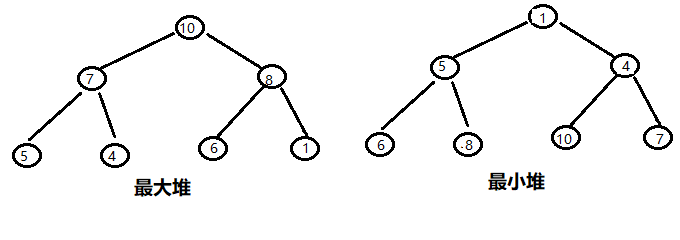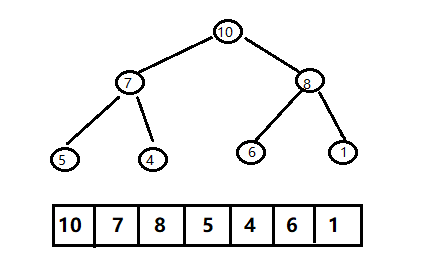1、建堆（升序建大堆，降序建小堆）；
2、交换数据；
3、向下调整。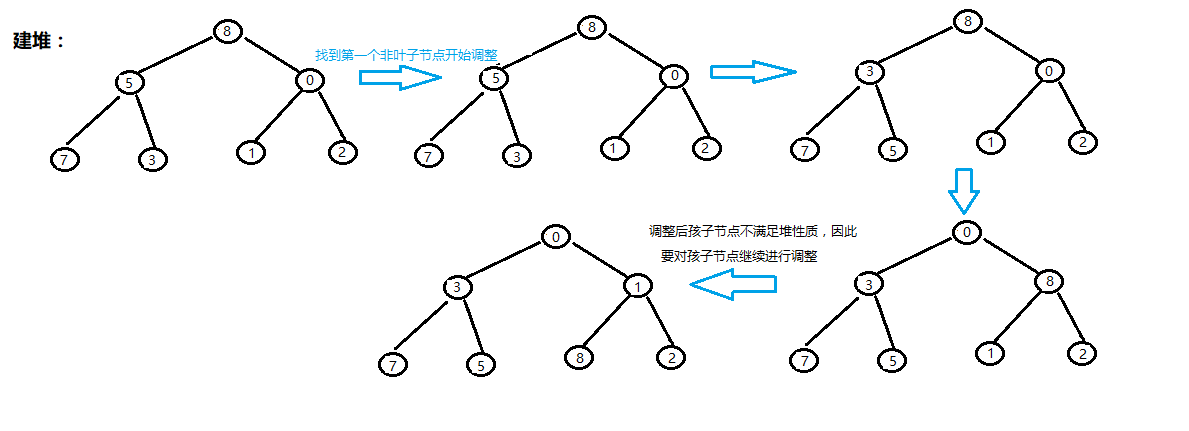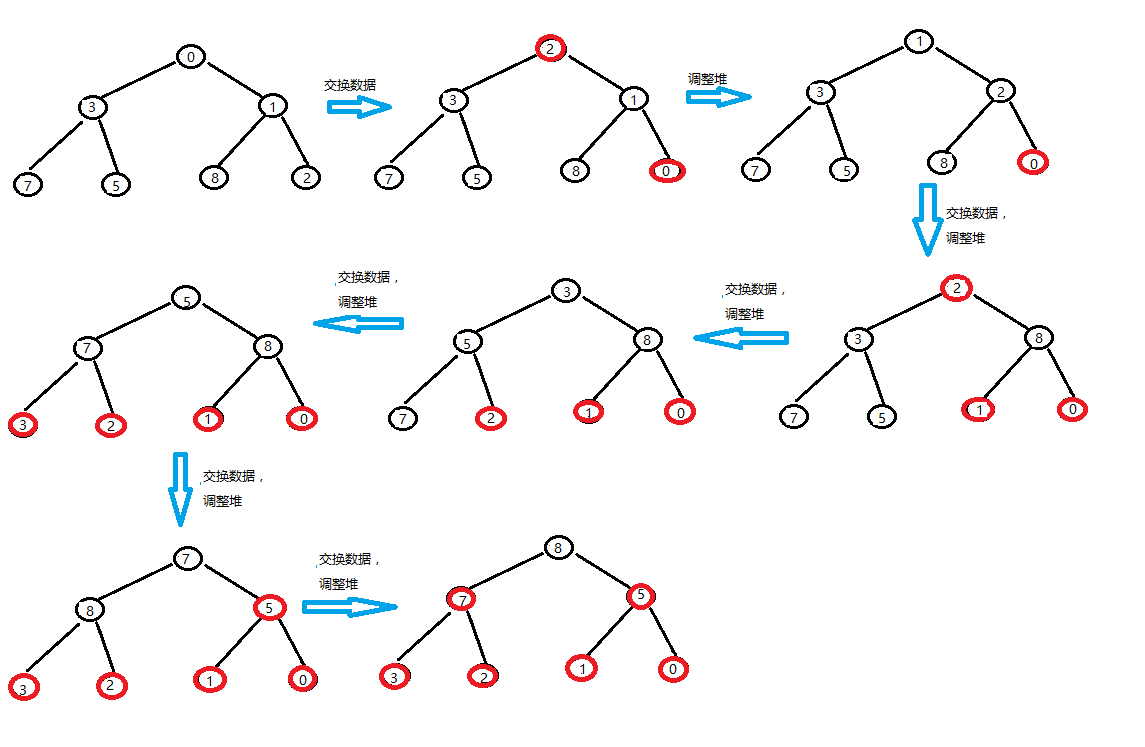``class Solution(object):#小顶堆，降序    def HeapAdjust(self, A, i, n):#删除        tmp = A[i]#i表示当前节点开始调整，主要是通用性        index = 2*i+1        while index < n:            if index+1 < n and A[index+1] < A[index]:#找到左右儿子最小值的索引                index += 1            if tmp < A[index]:#满足该条件时说明原始堆有序                break            #将最小儿子上移动            A[i] = A[index]            i = index            index = 2*i+1        A[i] = tmp#temp一直没有变，而且用来作为比较的参考值        print(A)    def ConstructMinHeap(self, A, n):#构建小根堆        #叶子节点不用参与重组，相当于是已经建好的堆        for i in range(n/2-1, -1, -1):            self.HeapAdjust(A, i, n)    def HeapSort(self, A):        #第一次将A与A[n - 1]交换，再对A[0…n-2]重新恢复堆,        #第二次将A与A[n – 2]交换，再对A[0…n - 3]重新恢复堆，        #重复这样的操作直到A与A交换。        self.ConstructMinHeap(A, len(A))#构建        for i in range(len(A)-1, 0, -1):            tmp = A[i]            A[i] = A            A = tmp            self.HeapAdjust(A, 0, i)        return A``

第一步：对无序的数组构造大根堆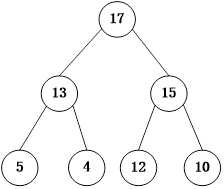第二步

将A与A互换，此时A为序列的最大值，A已经排序完毕，剩余的元素A~A形成新的未排序序列，由于此时序列不是大根堆，需要重构大根堆。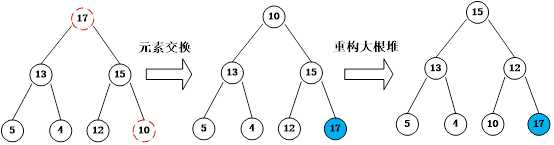第三步

将A与A互换，此时A为序列的最大值，A已经排序完毕，剩余的元素A~A形成新的未排序序列，由于此时序列不是大根堆，需要重构大根堆。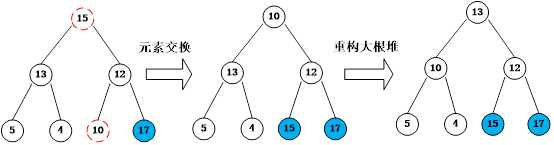第四步

将A与A互换，此时A为序列的最大值，A已经排序完毕，剩余的元素A~A形成新的未排序序列，由于此时序列不是大根堆，需要重构大根堆。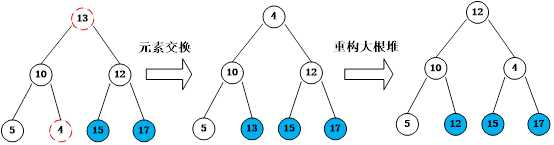第五步

将A与A互换，此时A为序列的最大值，A已经排序完毕，剩余的元素A~A形成新的未排序序列，由于此时序列不是大根堆，需要重构大根堆。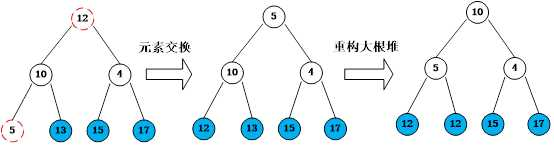第六步

将A与A互换，此时A为序列的最大值，A已经排序完毕，由于此时未排序的序列只剩下两个元素，而且A>A，将A与A互换即可得到最终的已排序序列。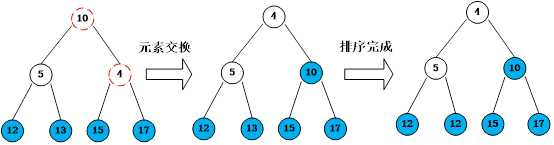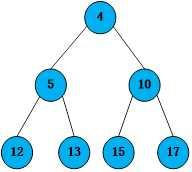``class Solution2(object):#大顶堆，升序    def HeapAdjust(self, A, i, n):        tmp = A[i]        index = 2*i+1#左右孩子的节点分别为2*i+1,2*i+2        while index <= n:            #选择出左右孩子较小的下标            if index < n and A[index] < A[index+1]:                index += 1            if tmp >= A[index]:#已经为大顶堆，=保持稳定性                break            A[i] = A[index]#将子节点上移            i = index#下一轮筛选            index *= 2#右孩子的节点        A[i] = tmp#temp一直没有变，插入正确的位置        print(A)    def ConstructMaxHeap(self, A, n):#构建大根堆        #叶子节点不用参与重组，相当于是已经建好的堆        for i in range(n/2-1, -1, -1):            self.HeapAdjust(A, i, n-1)    def HeapSort2(self, A):        #第一次将A与A[n - 1]交换，再对A[0…n-2]重新恢复堆,        #第二次将A与A[n – 2]交换，再对A[0…n - 3]重新恢复堆，        #重复这样的操作直到A与A交换。        self.ConstructMaxHeap(A, len(A))#构建        for i in range(len(A)-1, -1, -1):            tmp = A[i]            A[i] = A            A = tmp            self.HeapAdjust(A, 0, i-1)        return A``

7.归并排序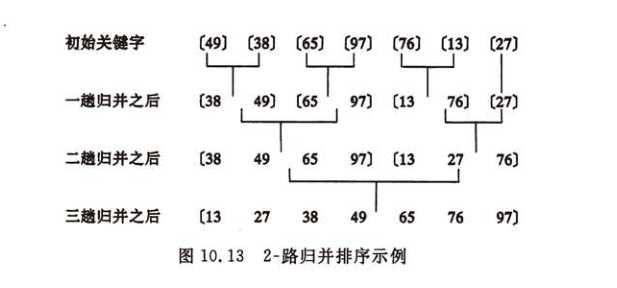``#/usr/bin/python#coding:-*-utf-8-*-class Solution(object):    def MergeSort(self, A):        left = 0        right = len(A)-1        self.MergeArray(A, left, right)        return A    def Merge(self, A, left, mid, right):        tmp = *(right-left+1)#len(A),初始化tmp中间数组        i = left        j = mid+1        k = 0        while i <= mid and j <= right:            if A[i] <= A[j]:                tmp[k] = A[i]                k += 1                i += 1            else:                tmp[k] = A[j]                k += 1                j += 1        print(A)        while i <= mid:            tmp[k] = A[i]            k += 1            i += 1 #tmp[k++] = A[i++],不会发生越界，因为i也是先赋值，再++；        while j <= right:            tmp[k] = A[j]            k += 1            j += 1        #将辅助空间内的数据转移到原始数组A        for p in range(len(tmp)):            A[left+p] = tmp[p]    def MergeArray(self, A, left, right):        if left >= right:            return        mid = (left+right)/2        self.MergeArray(A, left, mid)#左边        self.MergeArray(A, mid+1, right)#右边        self.Merge(A, left, mid, right)#合并``

1：复杂度

O(N^2)的有冒泡排序、插入排序、选择排序
O(N*logN)的有希尔排序、归并排序、快速排序、堆排序

2：空间复杂度

3：稳定性

(0)
(0)

0条